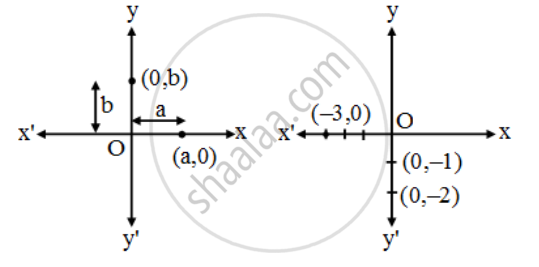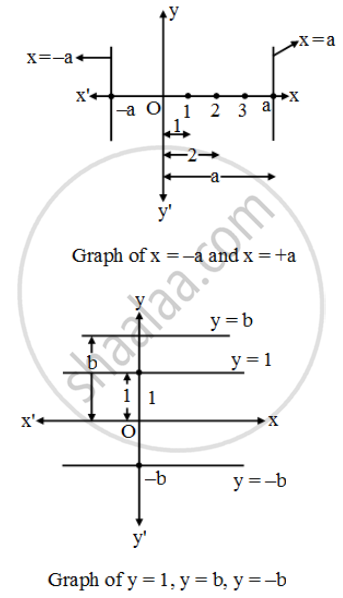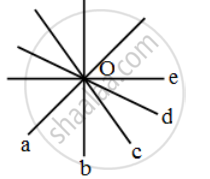# If (9/2, 6) is lies on graph of 4x + ky = 12 then find value of k - Mathematics

Sum

If (9/2, 6) is lies on graph of 4x + ky = 12 then find value of k

#### Solution

∵ x  =9/2 and y =6 are on the line

∴ put these value in given equation

4(9/2)+k(6) = 12

18+6k=12

6k=12-18

6k=-6

k =-1

Note:*
(1) Equation of x-axis is y = 0 and any point in ordered pair form which is on the x axis is (±a, 0).

(2) Equation of y axis is x = 0 and any point on y axis is (0, ±b)(3) Graph of line x = ±a is parallel to y axis

(4) Graph of line y = ±b is parallel to x axisConcurrent lines:
Three or more lines are called concurrent if all lines passes through a common point. These all lines a, b, c, d, e are passes through O.

∴ These are concurrent lines*Note:*
From a point there are infinite lines can pass, so we can find (or make) infinite equations of lines which passes through a given point.

Concept: Pair of Linear Equations in Two Variables
Is there an error in this question or solution?